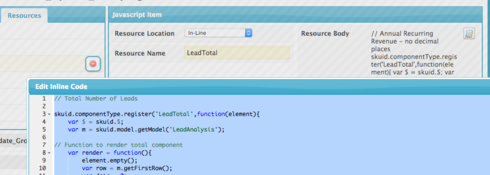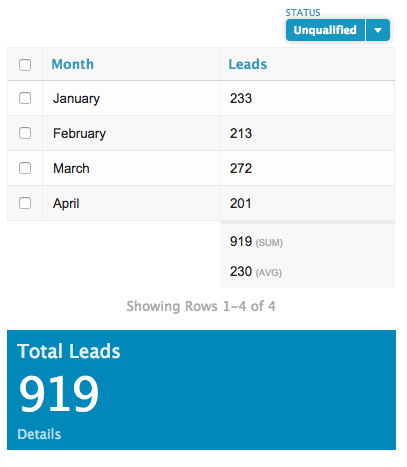# Dashboard with categories and totals that respond to filters.

edited September 1, 2016
Joe tagged onto another conversation but I thought his problem was worthy of a separate topic.   Here is his use case:
• I want to show a dashboard where information is aggregated by some category,  but there is also prominent display of the total.  (More prominent than the total line at the bottom of a table)

• I also want both the table of categorized data, and the prominent display of the total to respond to filters.
We first went down a multiple model approach,  but quickly discovered that the filters were going to be too complicated to keep everthing in synch.

What to do?
Tagged:

• Using a single model proved to be a better solution.   Here is what we did.

1. Create aggreage model to group data by the appropriate categories.

2. Build a custom component (with some custom CSS) to display the total.   I used work we described here:

3. Added a Javascript resource of type In-Line that iterates through the rows of the model and calculate the sum.Here is the code. Notice that I register 'LeadTotal' as my component name.  You need to make sure that name is used by the custom compoent created in step 2.
I'm using the "coundId" field from my aggregate model to do the calculation of totals.  You will need to make sure to use the right field alias from your model.
`// Total Number of Leads  skuid.componentType.register('LeadTotal',function(element){     var \$ = skuid.\$;     var m = skuid.model.getModel('LeadAnalysis');  // Function to render total component     var render = function(){         element.empty();         var row = m.getFirstRow();         var data = 0;              \$.each(m.data, function (i, row){              data += row.countId;          });                  data = addCommas(data);         var text =              "<a href='/apex/skuid__ui?page=MRR_Detail' class='metric'><div class= 'box ui-widget'><div class='kpi-title'> Total Leads </div><div class = 'kpi'>"             + data              +"</div><div class='bottomtext'>Details</div></div></a>";         element.append(             m.mergeRow(row,text)         );          };  // Runs render function when model is requeried by filters      skuid.events.subscribe('models.loaded',function(updateResult){        if (updateResult.models.LeadAnalysis)  {            render();        }     });  // Runs render function on initial page load.      render();      });      // Takes numbers and adds commas to them   var addCommas = function(str) {         str += '';         x = str.split('.');         x1 = x;         x2 = x.length > 1 ? '.' + x : '';         var rgx = /(d+)(d{3})/;         while (rgx.test(x1)) {             x1 = x1.replace(rgx, '\$1' + ',' + '\$2');         }         return x1 + x2;     };`
I've posted sample page XML here.  Copy it into a page in your org and you can see what is going on.

In addition,  there is code in this page XML to produce more elegant Month Names,  rather than just using numbers.   (Sweet)

Here is a picture.  Change the Status filter and both the table values and the total display are updated.